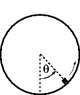# Mass Slides Inside a Hoop

• Becca93
In summary, the problem involves calculating the force with which a mass of 5.20E-1 kg pushes on a hoop with negligible friction, when the mass is at an angle of 27.0 degrees and has a speed of 5.27 m/s at the top. The solution requires considering both the force due to circular motion and the force of gravity acting on the mass. The correct answer is 29.59 N.

#### Becca93

Homework Statement

A mass M of 5.20E-1 kg slides inside a hoop of radius R=1.40 m with negligible friction. When M is at the top, it has a speed of 5.27 m/s. Calculate size of the force with which the M pushes on the hoop when M is at an angle of 27.0 degrees.

Picture attached at the bottom.

The attempt at a solution

E at the top of the circle should equal the energy at that particular part of the circle. So,

mg(2r) + (1/2)mvtop2 = mg(r-rcosθ) + (1/2)mv^2
m's cancel, so
g(2r) + (1/2)vtop2 = g(r-rcosθ) + (1/2)v^2
2(g(2r) + (1/2)vtop2 - g(r-rcosθ) = v^2

a = v^2/r

F = ma

When I plug everything in, I get
2(39.831) = v^2
v^2 = 79.66

a = 79.66/1.4
a = 56.90

F = (.52)(56.90)
F = 29.59 N

Please, can someone let me know where I'm going wrong?

#### Attachments

•prob25_1015hoop2.gif
1,008 bytes · Views: 613
Last edited:
You've calculated the force due to the circular motion, but what other force is also working on the mass?

gneill said:
You've calculated the force due to the circular motion, but what other force is also working on the mass?

Okay. I'm forgetting the force of gravity acting on the mass, aren't I?

Should I add mgcosθ to my answer? Is that all I'm missing?

Becca93 said:
Okay. I'm forgetting the force of gravity acting on the mass, aren't I?

Should I add mgcosθ to my answer? Is that all I'm missing?

That looks right. Since the hoop has "negligible friction", only the component of the force due to gravity that is normal to the hoop's surface should matter -- the other component acts to accelerate the mass tangentially.